# Assignments

In this topic we look at improper integrals. From the summary we learned that we have to use the mathematical topic limits which is no part of this website. Yet we do not expect any difficulties with this notion. Furthermore sometimes integration by parts is applied

1. Calculate: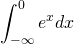Solution

2. Calculate: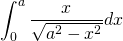Solution

3. Doest the following integral converge: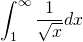Solution

4. Calculate: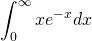Solution

5. Calculate: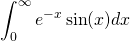Solution

0